Courses

# Taylor Series - Mathematical Methods of Physics, UGC - NET Physics Physics Notes | EduRev

## Physics for IIT JAM, UGC - NET, CSIR NET

Created by: Akhilesh Thakur

## Physics : Taylor Series - Mathematical Methods of Physics, UGC - NET Physics Physics Notes | EduRev

The document Taylor Series - Mathematical Methods of Physics, UGC - NET Physics Physics Notes | EduRev is a part of the Physics Course Physics for IIT JAM, UGC - NET, CSIR NET.
All you need of Physics at this link: Physics

Taylor series is a polynomial of infinite degree that can be used to represent many different functions, particularly functions that aren't polynomials. Taylor series have applications ranging from classical and modern physics to the computations that your handheld calculator makes when evaluating trigonometric expressions.

Taylor series are both useful and beautiful.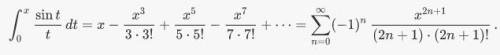Here, a Taylor series is being used to evaluate an integral that cannot be computed using known methods.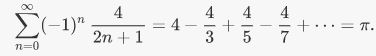Here, an elegant use of a Taylor series gives us an exact value  of π .

Introduction

Let f(x) be a real-valued function that is infinitely differentiable at x = x0 . The Taylor series expansion for the function f(x)  centered around the point x = x0 is given by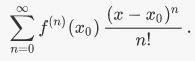Note that f(n) (x0)represents the  derivative of  at .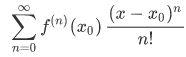It is not immediately obvious how this definition constructs a polynomial of infinite degree equivalent to the original function, f(x). Perhaps we can gain an understanding by writing out the first several terms of the Taylor series for f(x) = cos x centered at x = 0 . Note that there is nothing special about using x = 0 other than its ease in computation, but any other choice of center is allowed and will vary based on need.

We will now use the definition above to construct a graceful polynomial equivalency to cos x .

Because the formula for the Taylor series given in the definition above contains f(n) (x0) we should build a list containing the values of f(x) and its first four derivatives at x = 0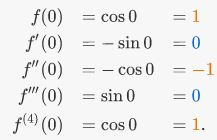We begin assembling the Taylor series by writing f(x) = [the first number in our list]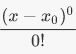like so: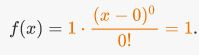So far, our constructed function f(x) = 1 looks nothing like f(x) = cos x. They merely have f(0) = 1 in common, but we shall add more terms. We add the next term from our list above, this time multiplied by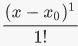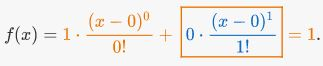Notice the exponent on (x - 0) and the argument inside the factorial are both 1 this time, rather than 0 as they were in the previous term. This is because the summation dictates that we increment n from 0 to 1. This process will continue by adding the next term from our list above, but again incrementing the power on  (x - 0) and the value inside the factorial.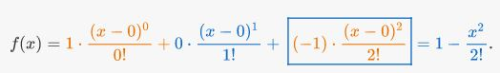Let's stop and look at what we have so far. After three terms, our Taylor series has given us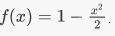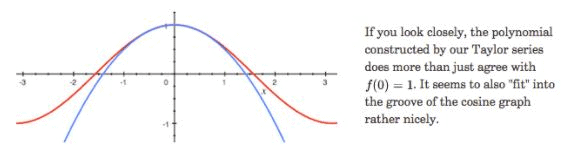Interestingly enough, if we continue taking numbers from our list while appending incremented powers of  and incremented factorials, then our Taylor series slowly but surely conforms to the cosine curve.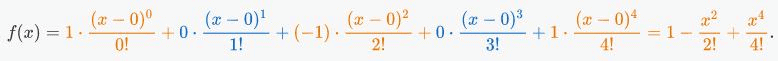At this point, we can guess at the emerging pattern. The powers on  are even, the factorials in the denominator are even, and the terms alternate signs. Note that more derivatives of the original function may be needed to discover a pattern, but only four derivatives were needed for this example. We encode this pattern into a summation which finally yields our Taylor series for cos x :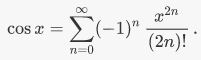In the animation below, each frame represents an additional term appended to the previous frame's Taylor series. As we add more terms, the Taylor series tends to fit better to the cosine function it's attempting to approximate.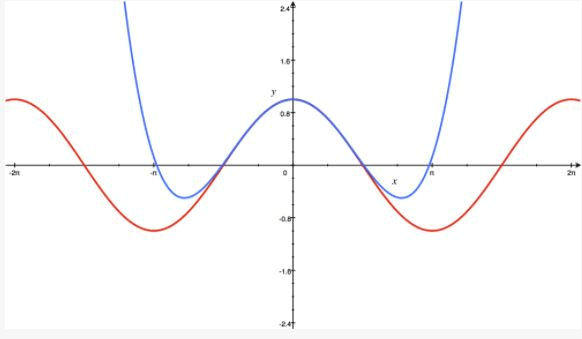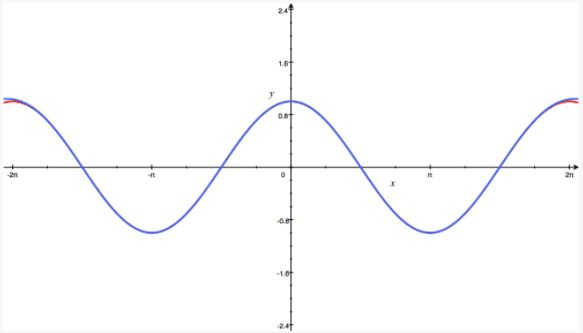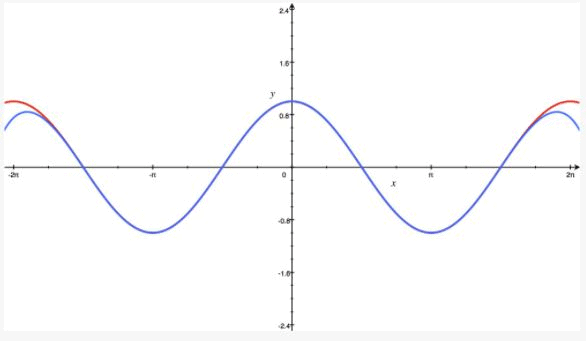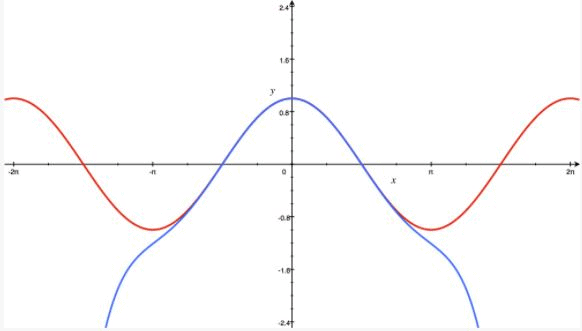Important note: Because this series expansion was centered at x = 0, this is also known as a Maclaurin series. A Maclaurin series is simply a Taylor series centered at x = 0 .

So how does this work exactly? What is the intuition for this formula? Let's solidify our understanding of Taylor series with a slightly more abstract demonstration. For the purposes of this next example, let T(x) represent the Taylor series expansion of f(x).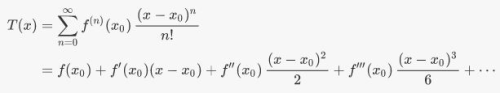It is important to note that the value of this summation at  x = x0 is simply f(x0), because all terms after the first will contain a 0 in their product. This means the value of the power series agrees with the value of the function at x0 (or that T(x0) = f(x0). Surely this is what we'd want from a series that purports to agree with the function! After all, if our claim is that the Taylor series T(x) equals the function f(x), then it should agree in value at x = x0. Granted, there are an uncountable number of other functions that share the same value at x0, so this equivalence is nothing special so far. Let's investigate by taking the derivative of the terms in the power series we have listed.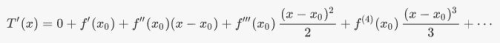If we evaluate the differentiated summation at  x = x0, then all terms after f'(x0)  vanish (again due to containing 0 in their product), leaving us with only f'(x0). So in addition to T(x0) = f(x0), we also have that T'(x0) = f'(x0), meaning the Taylor series and the function it represents agree in the value of their derivatives at . One can repeatedly differentiate T(x) and f(x) at x = x0 and find that this pattern continues. Indeed, the next derivative  T"(x0)  takes on the value f"(x0), the derivative after that T"'(x0)  takes on the value f"'(x0) and so on, all at x = x0.

This is a promising result! If we can ensure that the nth derivative T(x) of  agrees with the nth derivative of f(x) at x = x0 for all values of n, then we can expect the behavior of the Taylor series and f(x) to be identical.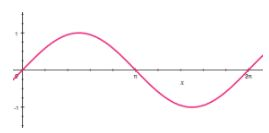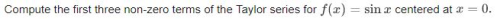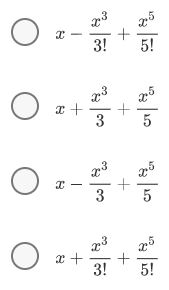There are already dozens of known Taylor series. Some of them are easy to derive on your own (and you should!) while others are far too complicated for the scope of this wiki.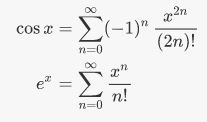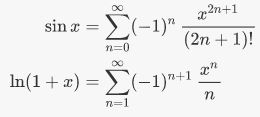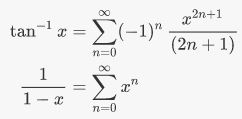The interval of convergence for a Taylor series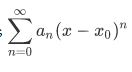is the set of values of x for which the series converges.

Examine the geometric power series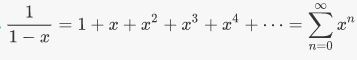Recall that a geometric progression of infinite terms

Taylor Polynomial Derivation

Suppose we want to interpolate an infinite number of points on the Cartesian plane using a continuous and differentiable function f. How can this be done?

Given n points on the Cartesian plane, the set of points can be interpolated using a polynomial of at least degree n - 1. Given an infinite number of points to interpolate, we need an infinite polynomial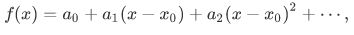where |x - x0| is within the radius of convergence.

Observation: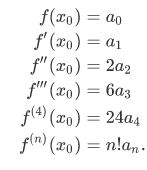Solving for each constant term expands the original function into the infinite polynomial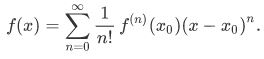Using Taylor Series in Approximations

Imagine that you have been taken prisoner and placed in a dark cell. Your captors say that you can earn your freedom, but only if you can produce an approximate value of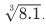. Worse than that, your approximation has to be correct to five decimal places! Even without a calculator in your cell, you can use the first few terms of the Taylor series for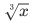about the point x = 8 as a tool for making a quick and decent approximation.

We certainly won't be able to compute an infinite number of terms in a Taylor series expansion for a function. However, as more terms are calculated in the Taylor series expansion of a function, the approximation of that function is improved.

Using the first three terms of the Taylor series expansion of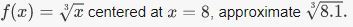We have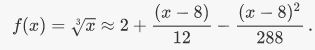The first three terms shown will be sufficient to provide a good approximation for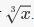Evaluating this sum at x = 8.1 gives an approximation for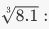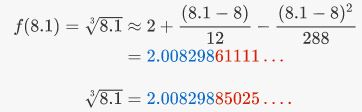With just three terms, the formula above was able to approximate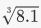to six decimal places of accuracy

159 docs

,

,

,

,

,

,

,

,

,

,

,

,

,

,

,

,

,

,

,

,

,

,

,

,

;